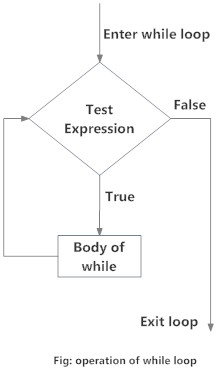# Python while Loop

Loops are used in programming to repeat a specific block of code. In this article, you will learn to create a while loop in Python.

## What is while loop in Python?

The while loop in Python is used to iterate over a block of code as long as the test expression (condition) is true.

We generally use this loop when we don't know beforehand, the number of times to iterate.

### Syntax of while Loop in Python

```while test_expression:
Body of while
```

In while loop, test expression is checked first. The body of the loop is entered only if the `test_expression` evaluates to `True`. After one iteration, the test expression is checked again. This process continues until the `test_expression` evaluates to `False`.

In Python, the body of the while loop is determined through indentation.

Body starts with indentation and the first unindented line marks the end.

Python interprets any non-zero value as `True`. `None` and `0` are interpreted as `False`.

### Flowchart of while Loop### Example: Python while Loop

```# Program to add natural
# numbers upto
# sum = 1+2+3+...+n

# To take input from the user,
# n = int(input("Enter n: "))

n = 10

# initialize sum and counter
sum = 0
i = 1

while i <= n:
sum = sum + i
i = i+1    # update counter

# print the sum
print("The sum is", sum)```

When you run the program, the output will be:

```Enter n: 10
The sum is 55
```

In the above program, the test expression will be `True` as long as our counter variable i is less than or equal to n (10 in our program).

We need to increase the value of counter variable in the body of the loop. This is very important (and mostly forgotten). Failing to do so will result in an infinite loop (never ending loop).

Finally the result is displayed.

## while loop with else

Same as that of for loop, we can have an optional `else` block with while loop as well.

The `else` part is executed if the condition in the while loop evaluates to `False`.

The while loop can be terminated with a break statement. In such case, the `else` part is ignored. Hence, a while loop's `else` part runs if no break occurs and the condition is false.

Here is an example to illustrate this.

```# Example to illustrate
# the use of else statement
# with the while loop

counter = 0

while counter < 3:
print("Inside loop")
counter = counter + 1
else:
print("Inside else")```

Output

```Inside loop
Inside loop
Inside loop
Inside else
```

Here, we use a counter variable to print the string `Inside loop` three times.

On the forth iteration, the condition in while becomes `False`. Hence, the `else` part is executed.